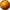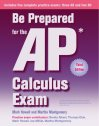Back to Be Prepared CalculusAbout this bookOrdering InfoOrder nowAP Calculus linksSkylight's home page## Appendix: Calculator Skills

 This material is provided to you as a supplement to the book Be Prepared for the AP Calculus Exam. You are not authorized to publish or distribute it in any form without our permission.  However, you may print out one copy of this chapter for personal use and for face-to-face teaching for each copy of the Be Prepared book that you own or receive from your school.

You should practice several calculator skills prior to the AP Exam.  The required skills are limited:

• produce a graph of a function within an arbitrary viewing window
• find the zeros of a function (that is, solve an equation numerically)
• calculate the derivative of a function at a given value
• calculate the value of a definite integral
Follow the lnks below to see how such types of problems are solved with different calculator models.

### HP Prime

There are other acceptable calculator methods to solve these problems.  If your calculator model does not match one of the models presented, consult your user’s manual to solve the examples.